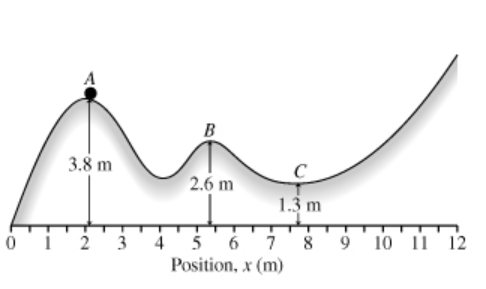# Problem: A particle slides along the frictionless track shown in the figure, starting at rest from point A.Find its speed at C.Express your answer using two significant figures.----------------------------

###### FREE Expert Solution

Conservation of energy:

Potential energy:

$\overline{){\mathbf{U}}{\mathbf{=}}{\mathbf{m}}{\mathbf{g}}{\mathbf{h}}}$

Kinetic energy:

$\overline{){\mathbf{K}}{\mathbf{E}}{\mathbf{=}}\frac{\mathbf{1}}{\mathbf{2}}{\mathbf{m}}{{\mathbf{v}}}^{{\mathbf{2}}}}$

KE0 = 0 (velocity at a is zero).

U0 = mghA

Work done by non-conservative forces, Wnc is zero.

Uf = mghC

83% (476 ratings)###### Problem DetailsA particle slides along the frictionless track shown in the figure, starting at rest from point A.

Find its speed at C.